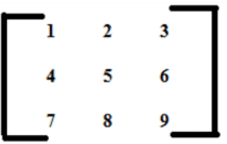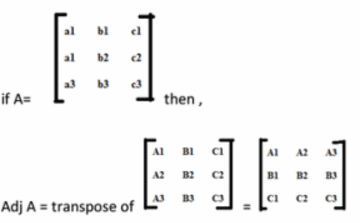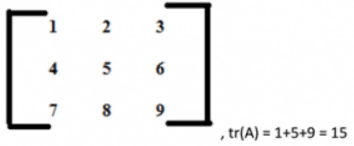# 1. Matrices, Linear Algebra, Engineering Mathematics, GATE | EduRev Notes

## Computer Science Engineering (CSE) : 1. Matrices, Linear Algebra, Engineering Mathematics, GATE | EduRev Notes

The document 1. Matrices, Linear Algebra, Engineering Mathematics, GATE | EduRev Notes is a part of the Computer Science Engineering (CSE) Course Mock Test Series - Computer Science Engg. (CSE) GATE.
All you need of Computer Science Engineering (CSE) at this link: Computer Science Engineering (CSE)

## Matrix Introduction

A matrix represents a collection of numbers arranged in an order of rows and columns. It is necessary to enclose the elements of a matrix in parentheses or brackets.
A matrix with 9 elements is shown below.This Matrix [M] has 3 rows and 3 columns. Each element of matrix [M] can be referred to by its row and column number. For example, a23=6

Order of a Matrix :
The order of a matrix is defined in terms of its number of rows and columns.
Order of a matrix = No. of rows ×No. of columns
Therefore Matrix [M] is a matrix of order 3 × 3.

Transpose of a Matrix :
The transpose [M]T of an m x n matrix [M] is the n x m matrix obtained by interchanging the rows and columns of [M].
if A= [aij] mxn , then AT = [bij] nxm where bij = aji

Properties of transpose of a matrix:

• (AT)TT = A
• (A+B)TT = ATT + BTT
• (AB)TT = BTTATT

Singular and Nonsingular Matrix:

1. Singular Matrix: A square matrix is said to be singular matrix if its determinant is zero i.e. |A|=0
2. Nonsingular Matrix: A square matrix is said to be non-singular matrix if its determinant is non-zero.

Properties of Matrix addition and multiplication:

1. A+B = B+A (Commutative)
2. (A+B)+C = A+ (B+C) (Associative)
3. AB ≠ BA (Not Commutative)
4. (AB) C = A (BC) (Associative)
5. A (B+C) = AB+AC (Distributive)

Square Matrix: A square Matrix has as many rows as it has columns. i.e. no of rows = no of columns.
Symmetric matrix: A square matrix is said to be symmetric if the transpose of original matrix is equal to its original matrix. i.e. (AT) = A.

Diagonal Matrix: A Symmetric matrix is said to be diagonal matrix where all the off diagonal elements are 0.
Identity Matrix: A diagonal matrix with 1s and only 1s on the diagonal. Identity matrix is denoted as I.
Orthogonal Matrix: A matrix is said to be orthogonal if AAT = ATA = I
Idemponent Matrix: A matrix is said to be idemponent if A2 = A
Involutary Matrix: A matrix is said to be Involutary if A2 = I.

Note: Every Square Matrix can uniquely be expressed as the sum of a symmetrix matrix and skew symmetric matrix. A = 1/2 (AT + A) + 1/2 (A – AT).2.

Inverse of a square matrix:

A-1 = Adj A / |A| ; |A|#0

Properties of inverse:

1. (A-1)-1 = A
2. (AB)-1 = B-1A-1
3. only a non singular square matrix can have an inverse.

Where should we use inverse matrix?

If you have a set of simultaneous equations:

7x + 2y + z = 21
3y – z = 5
-3x + 4y – 2x = -1

As we know when AX = B, then X = A-1B so we calculate inverse of A and by multiplying it B, we can get the values of x, y and z.

Trace of a matrix: trace of a matrix is denoted as tr(A) which is used only for square matrix and equals the sum of the diagonal elements of the matrix. For example:Offer running on EduRev: Apply code STAYHOME200 to get INR 200 off on our premium plan EduRev Infinity!

## Mock Test Series - Computer Science Engg. (CSE) GATE

131 docs|169 tests

,

,

,

,

,

,

,

,

,

,

,

,

,

,

,

,

,

,

,

,

,

,

,

,

,

,

,

,

,

,

;